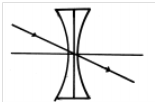# Lenses

A lens is a thin piece of a transparent material bounded by two spherical surfaces or by one spherical and other plane surface. The width or diameter of a lens is called the aperture of the lens. The geometric centre of a lens is known as its optic centre (O). The centre of curvature (C) is the centre of the sphere of which its surface forms a part. The radius of curvature (R) of a surface is the radius of the sphere of which the surface forms a part. The line passing through the centres of curvature of the two surfaces and optic centre of a lens is called the principal axis.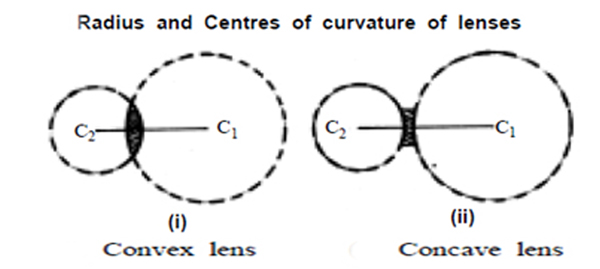When we place a comb in between the torch light and the convex lens and adjust the lens, we observe that the light rays converge at a point. In the case of concave lens, the rays appear to diverge from a point.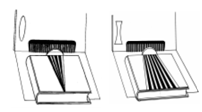A beam of rays parallel to the principal axis after refraction through the lens actually converges at a point on the principal axis. This point is called principal focus of a convex lens.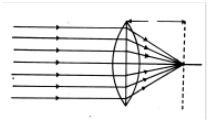A beam of rays parallel to the principal axis after refraction through a concave lens appear to diverge from a point on the principal axis. This point is called principal focus of a concave lens.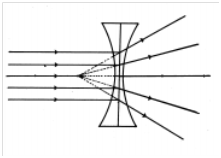The focal length of a lens is the distance between optic centre and principal focus of the lens.

#### Behavior of Rays in Convex Lens

An incident ray which is parallel to the principal axis, after refraction, passes through the principal focus on the other side of the lens.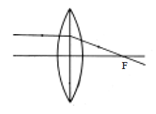An incident ray which passes through the principal focus, after refraction, emerges parallel to the principal axis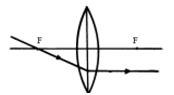An incident ray which passes through the optic centre goes straight without deflection.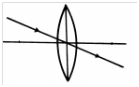#### Behavior of Rays in Concave Lenses

An incident ray which is parallel to the principal axis, after refraction, appears to diverge from the principal focus on the same side of the lens as the incident light.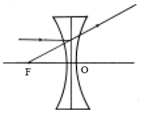An incident ray which proceeds towards the principal focus, after refraction, emerges parallel to the principal axis.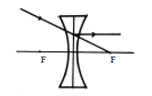An incident ray which passes through the optic centre goes straight without deflection.